Minima/ maxima (statement questions) - Geometry questions

Chapter 6 Class 12 Application of Derivatives
Concept wise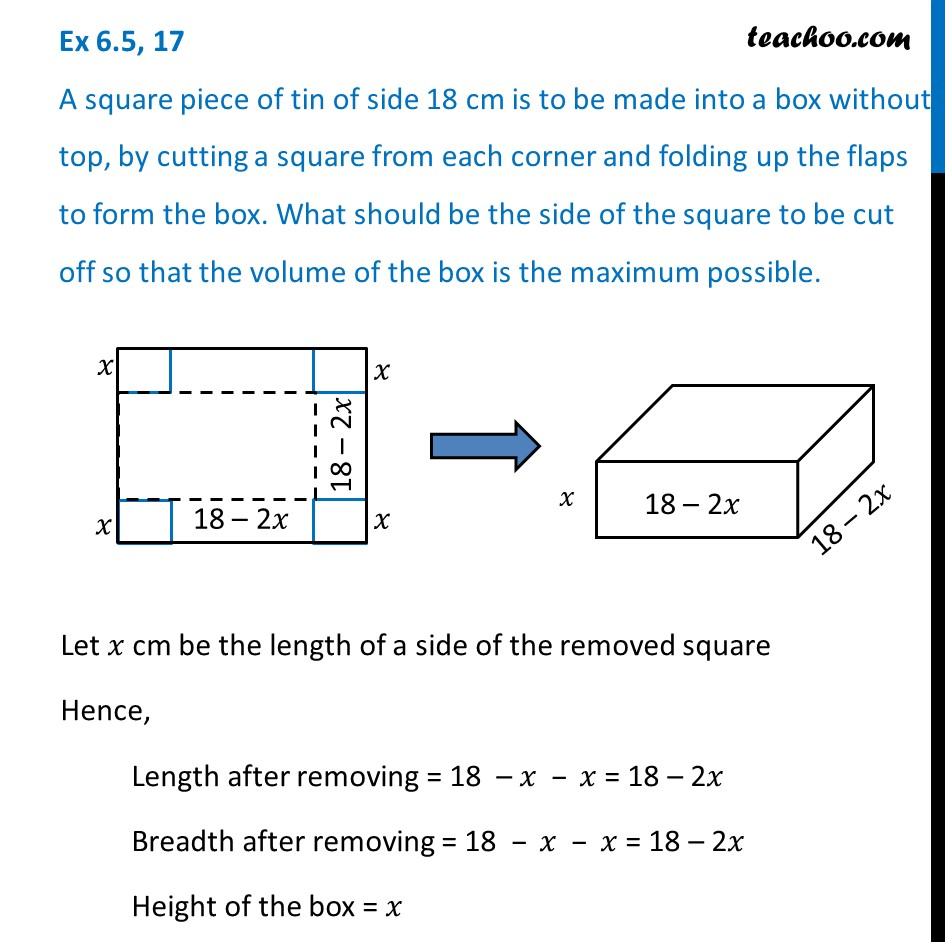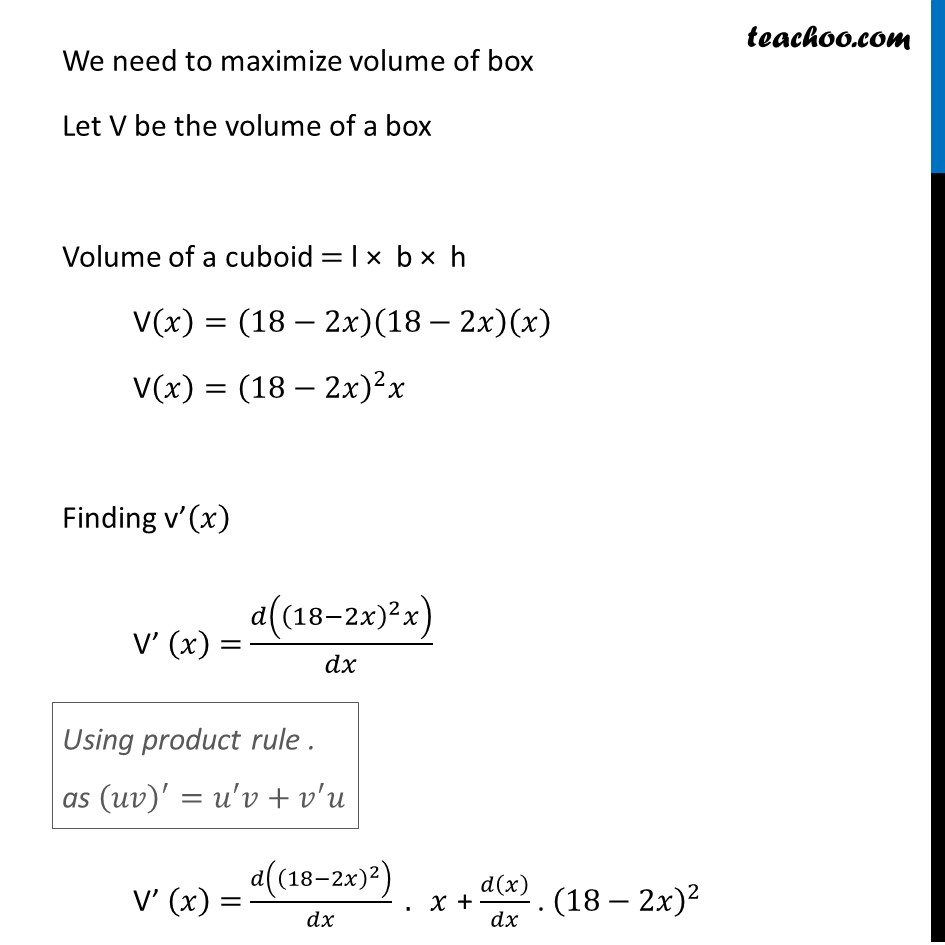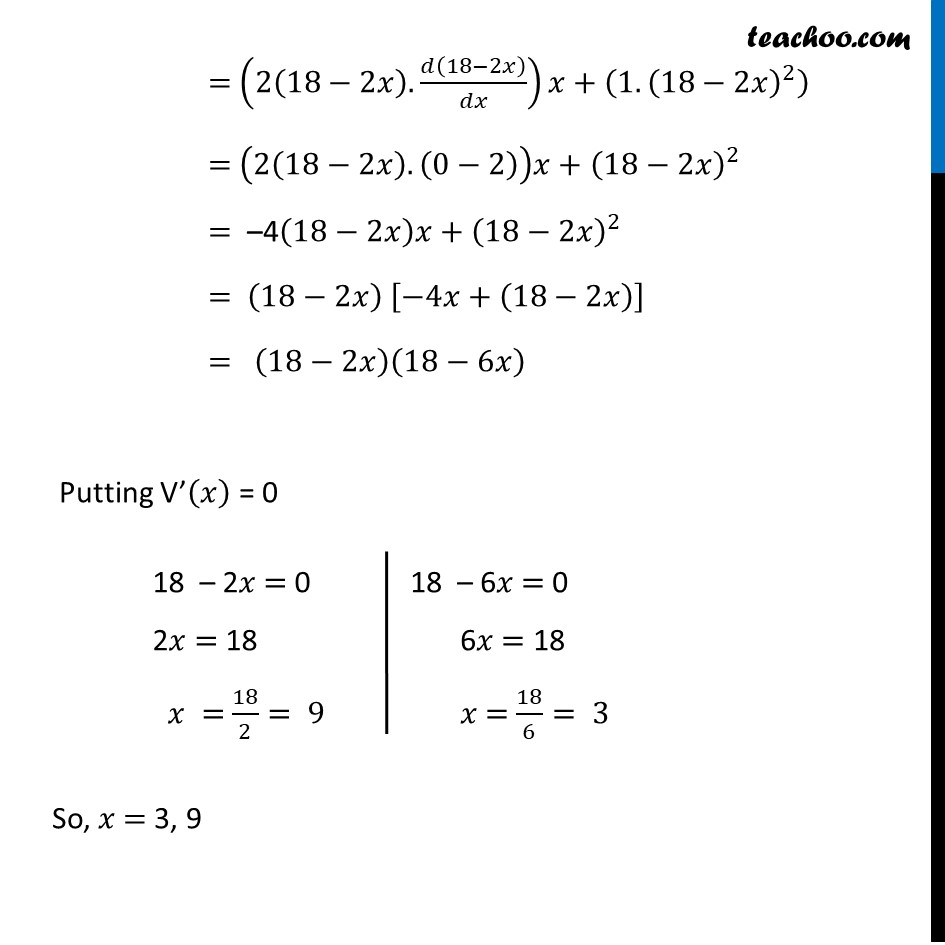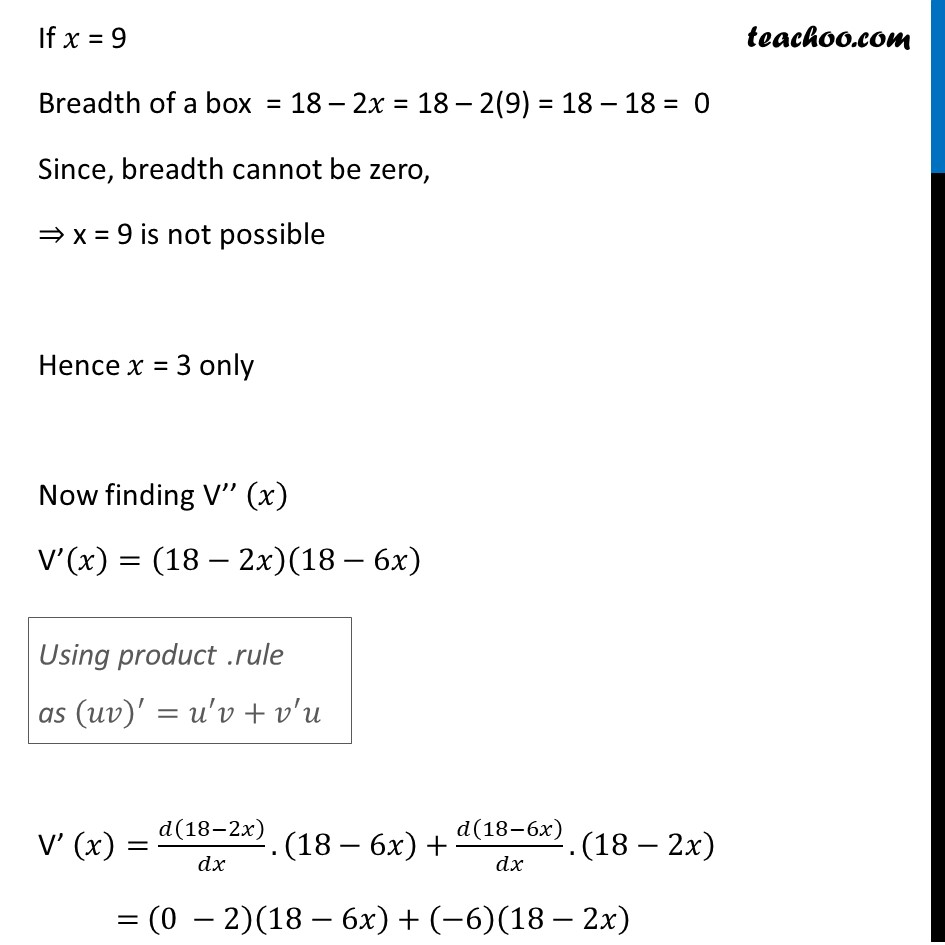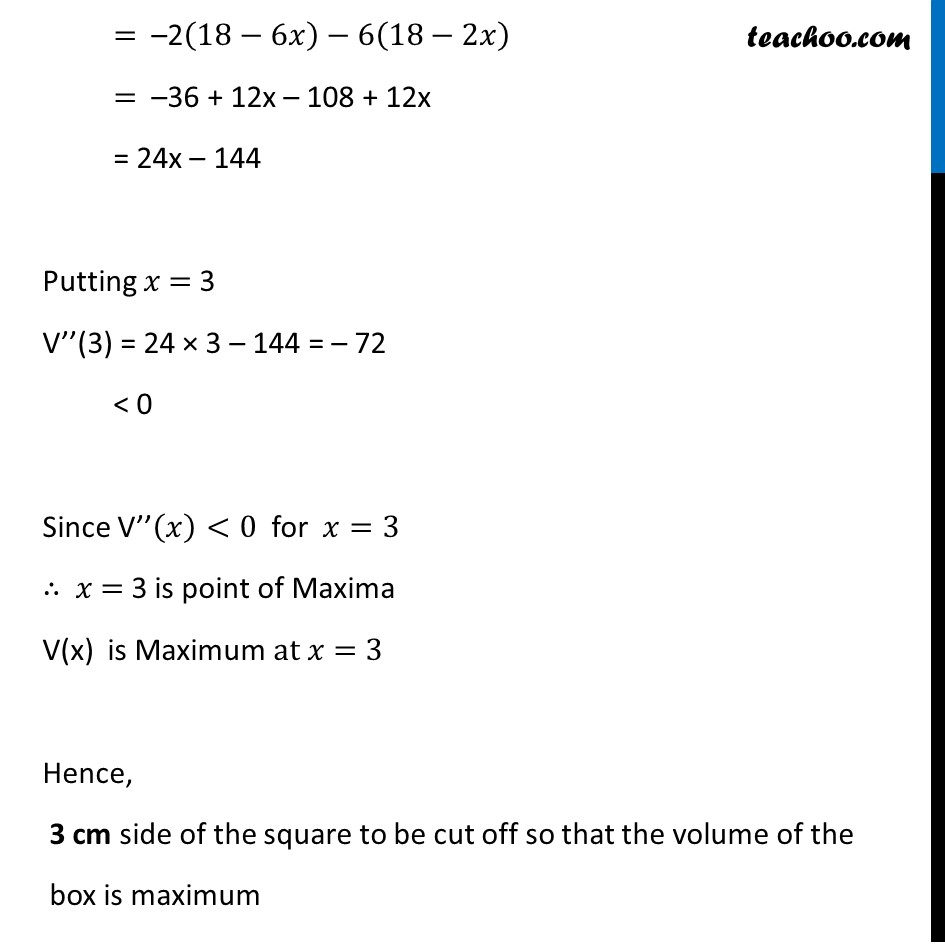Introducing your new favourite teacher - Teachoo Black, at only ₹83 per month

### Transcript

Ex 6.5, 17 A square piece of tin of side 18 cm is to be made into a box without top, by cutting a square from each corner and folding up the flaps to form the box. What should be the side of the square to be cut off so that the volume of the box is the maximum possible.Let 𝑥 cm be the length of a side of the removed square Hence, Length after removing = 18 – 𝑥 – 𝑥 = 18 – 2𝑥 Breadth after removing = 18 – 𝑥 – 𝑥 = 18 – 2𝑥 Height of the box = 𝑥 We need to maximize volume of box Let V be the volume of a box Volume of a cuboid = l × b × h V(𝑥)=(18−2𝑥)(18−2𝑥)(𝑥) V(𝑥)=(18−2𝑥)^2 𝑥 Finding v’(𝑥) V’ (𝑥) = 𝑑((18−2𝑥)^2 𝑥)/𝑑𝑥 V’ (𝑥) = 𝑑((18−2𝑥)^2 )/𝑑𝑥 . 𝑥 + 𝑑(𝑥)/𝑑𝑥 . (18−2𝑥)^2 Using product rule . as (𝑢𝑣)^′=𝑢^′ 𝑣+𝑣^′ 𝑢 = (2(18−2𝑥).𝑑(18−2𝑥)/𝑑𝑥)𝑥+(1.(18−2𝑥)^2 ) = (2(18−2𝑥).(0−2))𝑥+ (18−2𝑥)^2 = –4(18−2𝑥)𝑥+(18−2𝑥)^2 = (18−2𝑥) [−4𝑥+(18−2𝑥)] = (18−2𝑥)(18−6𝑥) Putting V’(𝑥) = 0 So, 𝑥 = 3, 9 18 – 2𝑥 = 0 2𝑥 = 18 𝑥 = 18/2= 9 18 – 6𝑥 = 0 6𝑥 = 18 𝑥 = 18/6= 3 If 𝑥 = 9 Breadth of a box = 18 – 2𝑥 = 18 – 2(9) = 18 – 18 = 0 Since, breadth cannot be zero, ⇒ x = 9 is not possible Hence 𝑥 = 3 only Now finding V’’ (𝑥) V’(𝑥)=(18−2𝑥)(18−6𝑥) V’ (𝑥)=𝑑(18−2𝑥)/𝑑𝑥.(18−6𝑥)+𝑑(18−6𝑥)/𝑑𝑥.(18−2𝑥) = (0 −2)(18−6𝑥)+(−6)(18−2𝑥) Using product .rule as (𝑢𝑣)^′=𝑢^′ 𝑣+𝑣^′ 𝑢 = –2(18−6𝑥)−6(18−2𝑥) = –36 + 12x – 108 + 12x = 24x – 144 Putting 𝑥 = 3 V’’(3) = 24 × 3 – 144 = – 72 < 0 Since V’’(𝑥)<0 for 𝑥=3 ∴ 𝑥 = 3 is point of Maxima V(x) "is Maximum " at 𝑥=3 Hence, 3 cm side of the square to be cut off so that the volume of the box is maximum v